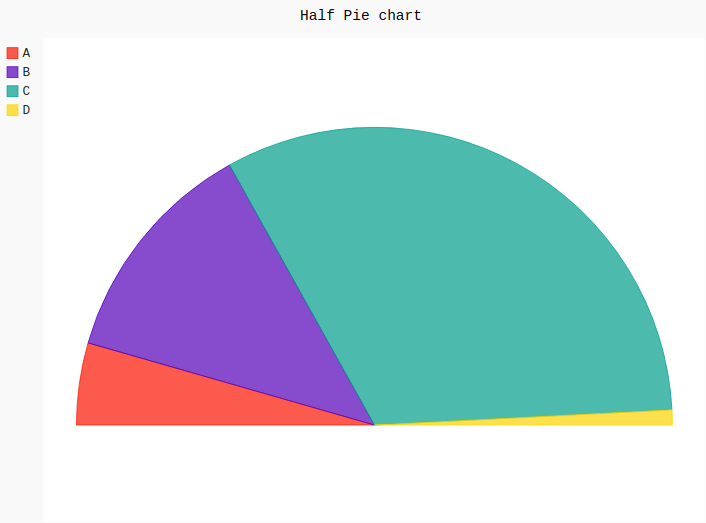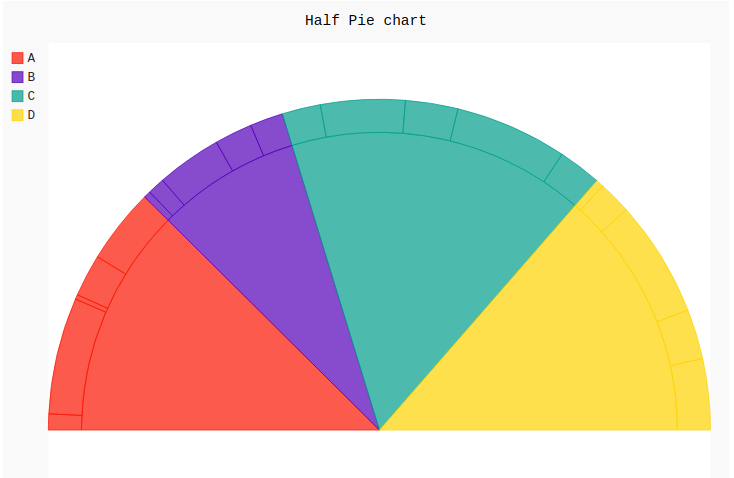GeeksforGeeks App
Open AppBrowser
Continue

## Related Articles

Pygal is a Python module that is mainly used to build SVG (Scalar Vector Graphics) graphs and charts. SVG is a vector-based graphics in the XML format that can be edited in any editor. Pygal can create graphs with minimal lines of code that can be easy to understand.

## Half Pie Chart

A half pie chart is also known as half-moon or semi-circle pie chart which is a 180-degree graph that represents the anatomy of a whole pie. The half pie chart works similarly to the regular pie chart. Its main advantage is that it takes less space in the layout and works like a full pie chart does. All the areas are plotted with different colors which helps to understand it more efficiently. It can be created using the `Pie()` method of pygal module by passing the half_pie attribute. This takes a boolean value.

Syntax:

`pie_chart = pygal.Pie(half_pie=True)`

Example 1:

 `# importing pygal``import` `pygal`` ` ` ` `# creating line chart object``pie_chart ``=` `pygal.Pie(half_pie ``=` `True``)`` ` `# naming the title``pie_chart.title ``=` `'Half Pie chart'`` ` ` ` `# random data``pie_chart.add(``'A'``, ``115``)``pie_chart.add(``'B'``, ``322``)``pie_chart.add(``'C'``, ``834``)``pie_chart.add(``'D'``, ``21``)`` ` `pie_chart`

Output:Example 2:

 `# importing pygal``import` `pygal``import` `numpy`` ` ` ` `# creating line chart object``pie_chart ``=` `pygal.Pie(half_pie ``=` `True``)`` ` `# naming the title``pie_chart.title ``=` `'Half Pie chart'`` ` ` ` `# Random data``pie_chart.add(``'A'``, numpy.random.rand(``5``))``pie_chart.add(``'B'``, numpy.random.rand(``5``))``pie_chart.add(``'C'``, numpy.random.rand(``5``))``pie_chart.add(``'D'``, numpy.random.rand(``5``))`` ` `pie_chart`

Output:My Personal Notes arrow_drop_up